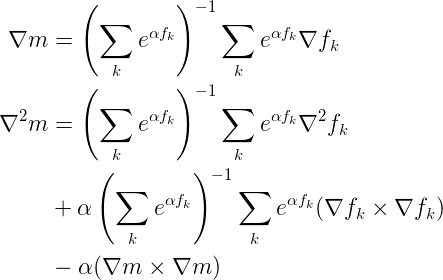# The Gradient and Hessian of the Smooth Max Over Functions

| Feedback

Suppose you have a set of functions over a vector space, and you are interested in taking the smooth-maximum over those functions. For example, maybe you are doing gradient descent, or convex optimization, etc, and you need a variant on “maximum” that has a defined gradient. The smooth maximum function has both a defined gradient and Hessian, and in this post I derive them.

I am using the logarithm-based definition of smooth-max, shown here:I will use the second variation above, ignoring function arguments, with the hope of increasing clarity. Applying the chain rule gives the ith partial gradient of smooth-max:Now that we have an ith partial gradient, we can take the jth partial gradient of that to obtain the (i,j)th element of a Hessian:This last re-grouping of terms allows us to see that we can express the full gradient and Hessian in the following more compact way:With a gradient and Hessian, we now have the tools we need to use smooth-max in algorithms such as gradient descent and convex optimization. Happy computing!